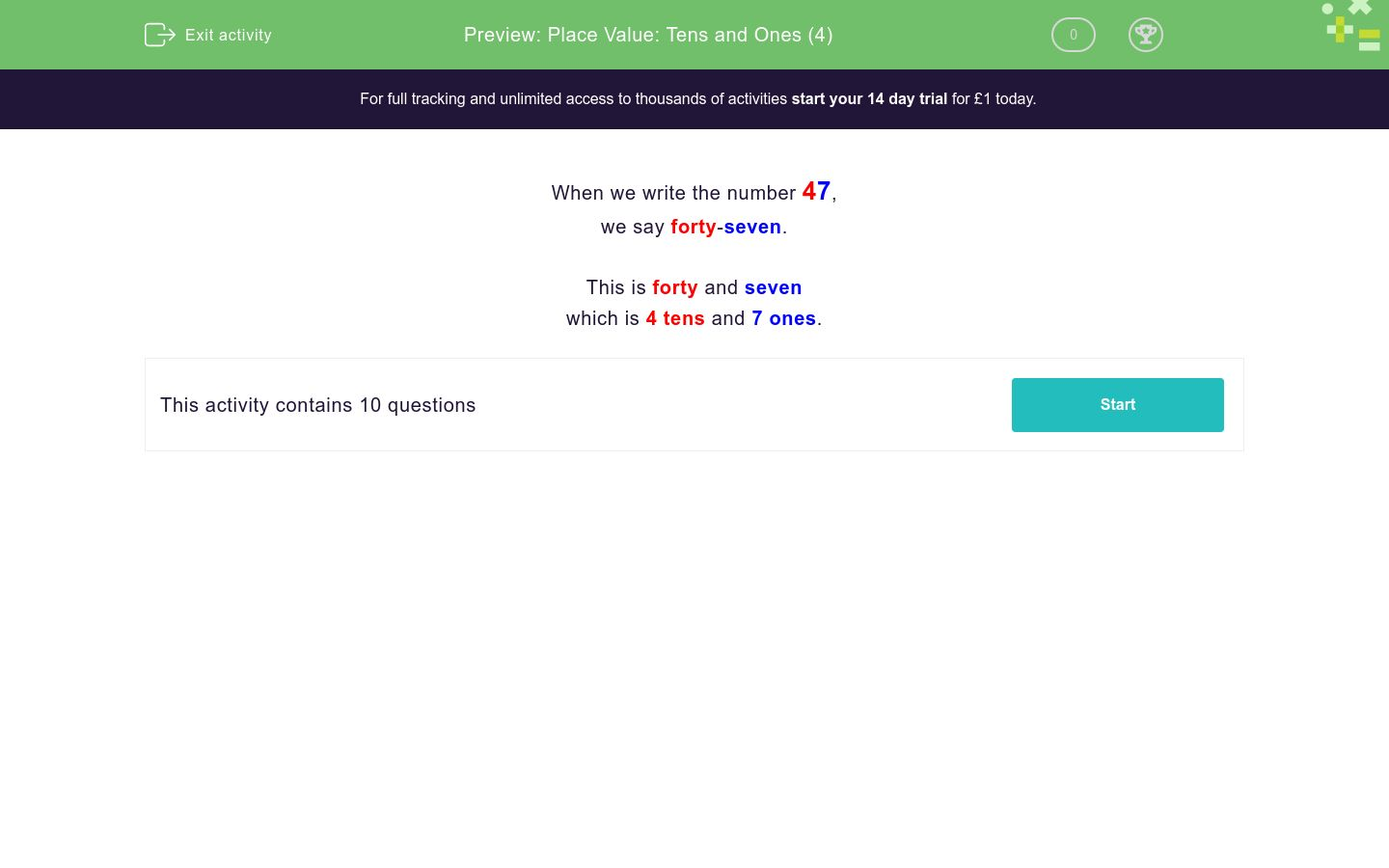# Place Value: Tens and Ones (4)

In this worksheet, students must match two-digit numbers with their equivalents split into tens and ones.Key stage:  KS 1

Curriculum topic:   Number: Number and Place Value

Curriculum subtopic:   Recognise Tens and Ones

Difficulty level:### QUESTION 1 of 10

When we write the number 47,

we say forty-seven.

This is forty and seven

which is 4 tens and 7 ones.

Match the numbers with their tens and ones.

## Column B

37
6 tens and 7 ones
47
3 tens and 7 ones
57
5 tens and 7 ones
67
4 tens and 7 ones

Match the numbers with their tens and ones.

## Column B

34
3 tens and 4 ones
44
5 tens and 4 ones
54
6 tens and 4 ones
64
4 tens and 4 ones

Match the numbers with their tens and ones.

## Column B

41
4 tens and 4 ones
44
4 tens and 6 ones
46
4 tens and 1 one
47
4 tens and 7 ones

Match the numbers with their tens and ones.

## Column B

21
2 tens and 8 ones
24
2 tens and 1 one
28
2 tens and 9 ones
29
2 tens and 4 ones

Match the numbers with their tens and ones.

## Column B

31
5 tens and 1 one
41
6 tens and 1 one
51
4 tens and 1 one
61
3 tens and 1 one

Match the numbers with their tens and ones.

## Column B

11
1 ten and 7 ones
14
1 ten and 1 one
16
1 ten and 6 ones
17
1 ten and 4 ones

Match the numbers with their tens and ones.

## Column B

12
5 tens and 3 ones
14
1 ten and 2 ones
36
3 tens and 6 ones
53
1 ten and 4 ones

Match the numbers with their tens and ones.

## Column B

30
3 tens and 0 ones
42
6 tens and 8 ones
59
4 tens and 2 ones
68
5 tens and 9 ones

Match the numbers with their tens and ones.

## Column B

50
2 tens and 2 ones
22
9 tens and 8 ones
57
5 tens and 0 ones
98
5 tens and 7 ones

Match the numbers with their tens and ones.

## Column B

75
5 tens and 7 ones
57
4 tens and 8 ones
84
8 tens and 4 ones
48
7 tens and 5 ones
• Question 1

Match the numbers with their tens and ones.

## Column B

37
3 tens and 7 ones
47
4 tens and 7 ones
57
5 tens and 7 ones
67
6 tens and 7 ones
EDDIE SAYS
In 37, 3 is the tens digit and 7 is the ones digit.
• Question 2

Match the numbers with their tens and ones.

## Column B

34
3 tens and 4 ones
44
4 tens and 4 ones
54
5 tens and 4 ones
64
6 tens and 4 ones
EDDIE SAYS
In 34, 3 is the tens digit and 4 is the ones digit.
• Question 3

Match the numbers with their tens and ones.

## Column B

41
4 tens and 1 one
44
4 tens and 4 ones
46
4 tens and 6 ones
47
4 tens and 7 ones
EDDIE SAYS
In 47, 4 is the tens digit and 7 is the ones digit.
• Question 4

Match the numbers with their tens and ones.

## Column B

21
2 tens and 1 one
24
2 tens and 4 ones
28
2 tens and 8 ones
29
2 tens and 9 ones
EDDIE SAYS
In 24, 2 is the tens digit and 4 is the ones digit.
• Question 5

Match the numbers with their tens and ones.

## Column B

31
3 tens and 1 one
41
4 tens and 1 one
51
5 tens and 1 one
61
6 tens and 1 one
EDDIE SAYS
In 31, 3 is the tens digit and 1 is the ones digit.
• Question 6

Match the numbers with their tens and ones.

## Column B

11
1 ten and 1 one
14
1 ten and 4 ones
16
1 ten and 6 ones
17
1 ten and 7 ones
EDDIE SAYS
In 14, 1 is the tens digit and 4 is the ones digit.
• Question 7

Match the numbers with their tens and ones.

## Column B

12
1 ten and 2 ones
14
1 ten and 4 ones
36
3 tens and 6 ones
53
5 tens and 3 ones
EDDIE SAYS
In 12, 1 is the tens digit and 2 is the ones digit.
• Question 8

Match the numbers with their tens and ones.

## Column B

30
3 tens and 0 ones
42
4 tens and 2 ones
59
5 tens and 9 ones
68
6 tens and 8 ones
EDDIE SAYS
In 30, 3 is the tens digit and 0 is the ones digit.
• Question 9

Match the numbers with their tens and ones.

## Column B

50
5 tens and 0 ones
22
2 tens and 2 ones
57
5 tens and 7 ones
98
9 tens and 8 ones
EDDIE SAYS
In 50, 5 is the tens digit and 0 is the ones digit.
• Question 10

Match the numbers with their tens and ones.

## Column B

75
7 tens and 5 ones
57
5 tens and 7 ones
84
8 tens and 4 ones
48
4 tens and 8 ones
EDDIE SAYS
In 75, 7 is the tens digit and 5 is the ones digit.
---- OR ----